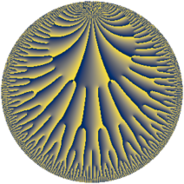# Properties

 Label 25.34.eLevel $25$ Weight $34$ Character orbit 25.e Rep. character $\chi_{25}(4,\cdot)$ Character field $\Q(\zeta_{10})$ Dimension $328$ Sturm bound $85$

# Related objects

## Defining parameters

 Level: $$N$$ $$=$$ $$25 = 5^{2}$$ Weight: $$k$$ $$=$$ $$34$$ Character orbit: $$[\chi]$$ $$=$$ 25.e (of order $$10$$ and degree $$4$$) Character conductor: $$\operatorname{cond}(\chi)$$ $$=$$ $$25$$ Character field: $$\Q(\zeta_{10})$$ Sturm bound: $$85$$

## Dimensions

The following table gives the dimensions of various subspaces of $$M_{34}(25, [\chi])$$.

Total New Old
Modular forms 336 336 0
Cusp forms 328 328 0
Eisenstein series 8 8 0

## Trace form

 $$328q - 5q^{2} - 5q^{3} + 352187318269q^{4} + 897601920255q^{5} - 5659399684099q^{6} + 3919070765935810q^{8} + 155032143469110191q^{9} + O(q^{10})$$ $$328q - 5q^{2} - 5q^{3} + 352187318269q^{4} + 897601920255q^{5} - 5659399684099q^{6} + 3919070765935810q^{8} + 155032143469110191q^{9} + 22271193818331875q^{10} - 392543399750177989q^{11} - 3752295944767406085q^{12} - 5q^{13} + 18409493125371526783q^{14} - 103325648847865866225q^{15} - 1784507369696420215607q^{16} + 208630128565519263515q^{17} + 603918334415854145485q^{19} + 10557009978644257265475q^{20} - 15774370344809451307494q^{21} - 62565678060538208787850q^{22} + 50913937092996652695755q^{23} - 214884463941084331340720q^{24} + 369630814700805786581715q^{25} + 1392568700124137209776786q^{26} + 2404609885857682269828595q^{27} - 4508872899196069095669765q^{28} + 1787422073667397982532745q^{29} - 19707319271742636358793525q^{30} + 6440819317072818189184161q^{31} - 43332094907530000222549765q^{33} + 40618697017218950293307373q^{34} - 26285894304203756723954130q^{35} - 733444318837595807767423827q^{36} + 454267796000171232458895630q^{37} - 439434997994244011879776185q^{38} - 16530761168031563647755833q^{39} - 928701119265102370478495590q^{40} - 220199118925265662731774109q^{41} - 4777837380334807111062805315q^{42} - 4814450630372787414415944452q^{44} - 2454722165353234833379956855q^{45} - 14530299338609028002865059039q^{46} + 6543416761795282936012151025q^{47} - 2707004419260298696030253430q^{48} - 334909983302301911040683570956q^{49} - 28158704351734508754992367915q^{50} + 19367926877179854098166762906q^{51} - 192244190650431484366321105260q^{52} + 54173851052621193237449639540q^{53} + 23198025460755550969604529665q^{54} - 170453067448553170614579648475q^{55} - 7445090376389891713181726960q^{56} - 251237533752802121725384761430q^{58} + 74689935521459956100735927895q^{59} - 2595079013886305830034963307880q^{60} - 161897865606021230530318028859q^{61} - 311552648545962692881572345480q^{62} + 95593078655174897547297944610q^{63} + 9381831760640979270874481875404q^{64} - 1715181756840796859618113740610q^{65} - 3423218455244934429645398834318q^{66} + 128769211920487187037788437795q^{67} + 2052583968908209317916090745987q^{69} - 3712393741033818216539550163290q^{70} - 1331035528097329277114600707719q^{71} + 63047568606735557713452890939855q^{72} + 6130549211927977885326144800515q^{73} - 78831138764066445426570309462252q^{74} + 56154667810354755087485176024565q^{75} + 104094823992840106153154516675120q^{76} - 31869406199015943996284997121490q^{77} - 151147503385452293859505899173225q^{78} + 77948888580679597722934213075175q^{79} + 118375255367113239140845408980650q^{80} - 45071593461186833790635739471687q^{81} + 173824201460495341611564831029055q^{83} - 667286297341271192226211420462062q^{84} + 525278091710734023860228880866840q^{85} - 759969567850007046672226801727109q^{86} - 584507700005654370909048029428045q^{87} - 120978417895322407075725927335170q^{88} - 402611860413403213044079810271950q^{89} - 438006510389248435686022430475265q^{90} - 833380852148569239280599836950574q^{91} - 730271007384074229103991997749670q^{92} + 1498468250847743527153037549810073q^{94} - 975663823503333512718440049885735q^{95} - 450097968787843477006636639749304q^{96} + 4527675954202041727822942189058365q^{97} + 3176663995694595945047030706857540q^{98} - 6741192097917340633886228296954728q^{99} + O(q^{100})$$

## Decomposition of $$S_{34}^{\mathrm{new}}(25, [\chi])$$ into newform subspaces

The newforms in this space have not yet been added to the LMFDB.